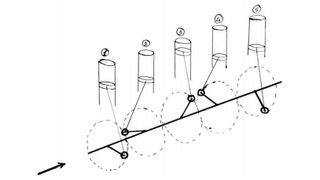###### Numerical - Multi Cylinder Inline Engine (with firing order)
Basic knowledge about Firing Order The firing order is a sequence of giving spark/firing to the cylinders of multi-cylinder inline engines…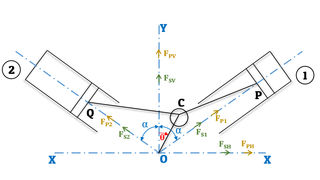###### Balancing of V-Engines
V-Engine A V-engine is a two cylinder engine, which has a common crank and the axis of cylinder makes a "V" shape. Since V-engines have a…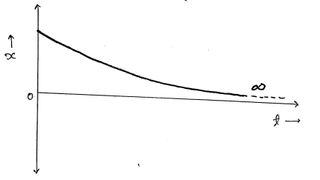###### Over-Damped System (​ξ>1)
We know that the characteristic equation of the damped free vibration system is, This is a quadratic equation having two roots and ; In…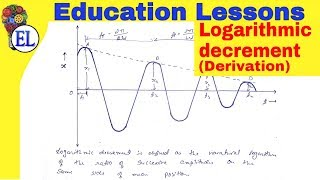###### Logarithmic Decrement (​δ)
Logarithmic decrement Logarithmic decrement is defined as the natural logarithm of the ratio of successive amplitude on the same side of…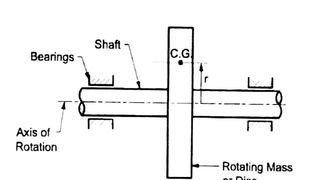###### Static and Dynamic Balancing
When machine is in working condition different forces are acting on it which may cause machine to vibrate and cause damage to machine parts…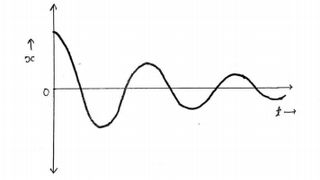###### Under-Damped System (​ξ < 1)
Underdamped system If the damping factor is less than one or the damping coefficient is less than critical damping coefficient , then…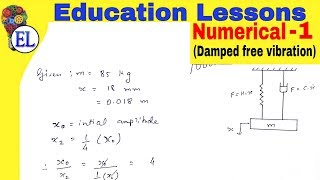###### Damped free Vibration - Numerical 1
A mass of 85 kg is supported on a spring which deflects 18 mm under the weight of the mass. The vibrations of the mass are constrained to be…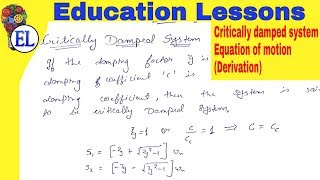###### Critically Damped System (ξ = 1)
Critically damped system(ξ=1): If the damping factor ξ is equal to one, or the damping coefficient c is equal to critical damping…